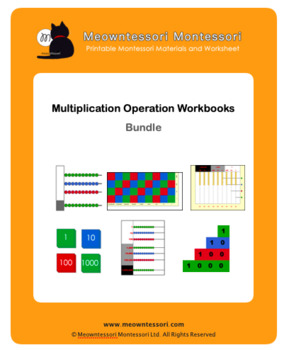# Montessori Multiplication Operation Workbooks BundleSubject
Resource Type
Format
Zip (28 MB)
\$98.80
Bundle
List Price:
\$120.00
You Save:
\$21.20
\$98.80
Bundle
List Price:
\$120.00
You Save:
\$21.20

#### Products in this Bundle (20)

showing 1-5 of 20 products

### Description

This Montessori Multiplication Operation Workbooks Bundle contains following products:

• Stamp Game Multiplication (Static)
• Stamp Game Multiplication (Dynamic)
• Dot Game Multiplication
• Small Bead Frame Multiplication (Static)
• Small Bead Frame Multiplication (Dynamic)
• Large Bead Frame Multiplication (1-digit multiplier)
• Large Bead Frame Multiplication (2-digit multiplier)
• Large Bead Frame Multiplication (3-digit multiplier)
• Checkerboard Introduction
• Checkerboard Multiplication (1-digit multiplier)
• Checkerboard Multiplication (2-digit multiplier)
• Checkerboard Multiplication (3-digit multiplier)
• Golden Bead Frame Multiplication (1-digit multiplier)
• Golden Bead Frame Multiplication (2-digit multiplier)
• Golden Bead Frame Multiplication (3-digit multiplier)
• Bank Game Introduction
• Bank Game Multiplication (1-digit multiplier)
• Bank Game Multiplication (2-digit multiplier)
• Bank Game Multiplication (3-digit multiplier)
• Abstract Multiplication Practice (1, 2, 3-digit multipliers)
Total Pages
N/A
N/A
Teaching Duration
N/A
Report this Resource to TpT
Reported resources will be reviewed by our team. Report this resource to let us know if this resource violates TpT’s content guidelines.